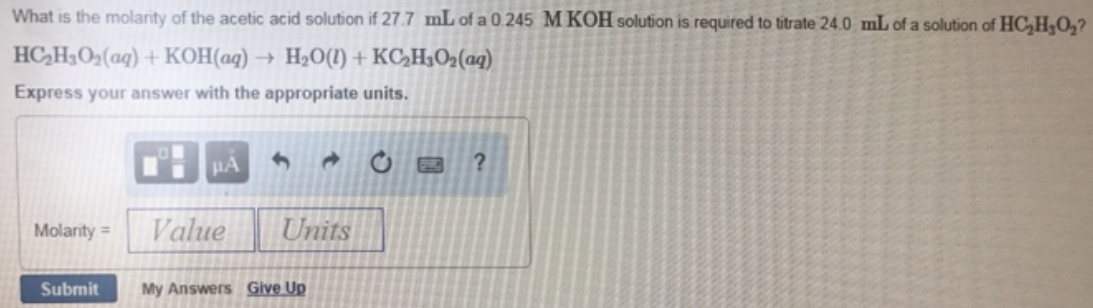# Problem: What is the molarity of the acetic acid solution if 27.7 mL of a 0.245 M KOH solution is required to titrate 24.0 mL of a solution of HC2H3O2? HC2H3O2 (aq) + KOH (aq) → H2O (l) + KC2H3O2 (aq) Express your answer with the appropriate units.

###### FREE Expert Solution
100% (324 ratings)###### Problem Details

What is the molarity of the acetic acid solution if 27.7 mL of a 0.245 M KOH solution is required to titrate 24.0 mL of a solution of HC2H3O2

HC2H3O2 (aq) + KOH (aq) → H2O (l) + KC2H3O2 (aq)